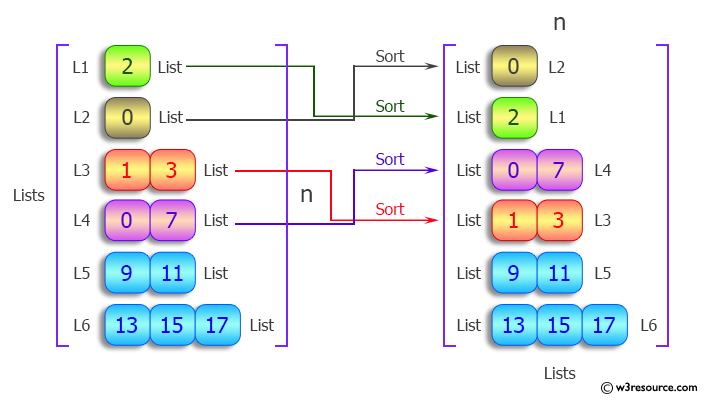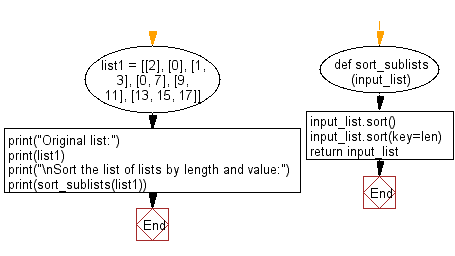﻿ Python: Sort a list of lists by length and value - w3resource# Python: Sort a list of lists by length and value

## Python List: Exercise - 96 with Solution

Write a Python program to sort a given list of lists by length and value.

Sample Solution:

Python Code:

``````def sort_sublists(input_list):
input_list.sort()  # sort by sublist contents
input_list.sort(key=len)
return input_list

list1 = [, , [1, 3], [0, 7], [9, 11], [13, 15, 17]]
print("Original list:")
print(list1)
print("\nSort the list of lists by length and value:")
print(sort_sublists(list1))
```
```

Sample Output:

```Original list:
[, , [1, 3], [0, 7], [9, 11], [13, 15, 17]]

Sort the list of lists by length and value:
[, , [0, 7], [1, 3], [9, 11], [13, 15, 17]]
```

Pictorial Presentation:Flowchart:## Visualize Python code execution:

The following tool visualize what the computer is doing step-by-step as it executes the said program:

Python Code Editor:

Have another way to solve this solution? Contribute your code (and comments) through Disqus.

What is the difficulty level of this exercise?

Test your Python skills with w3resource's quiz

﻿

## Python: Tips of the Day

Floor Division:

When we speak of division we normally mean (/) float division operator, this will give a precise result in float format with decimals.

For a rounded integer result there is (//) floor division operator in Python. Floor division will only give integer results that are round numbers.

```print(1000 // 300)
print(1000 / 300)```

Output:

```3
3.3333333333333335```